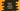# Python string isalnum method explanation with example## Python string isalnum() method:

Python string isalnum() method is used to check if a string contains alphanumeric characters. This is an inbuilt function of Python string. This method returns one boolean value.

Let’s learn how to use this method with examples.

### isalnum() method definition:

isalnum() method is defined as like below:

``str.isalnum()``

It doesn’t take any parameter and it returns one boolean value. str is the string on which we are calling this method.

### Return value of isalnum():

isalnum() returns one boolean value.

• True if all characters of the string str are alphanumeric.
• False if at least one character of the string str is not alphanumeric.

### Example of isalnum() to check if a string is alphanumeric:

Let’ take an example of isalnum:

``````given_str_list = ['hello', '1hello', 'hello world', 'helloworld',
'hello world !!', 'helloworld!!', '1234', '1 2 3 4', '@', '', '   ']

for str in given_str_list:
print(f'{str} => {str.isalnum()}')``````

In this example, given_str_list includes different types of strings. The for loop iterates over the strings of the list and runs isalnum on each string. It will print the below output:

``````hello => True
1hello => True
hello world => False
helloworld => True
hello world !! => False
helloworld!! => False
1234 => True
1 2 3 4 => False
@ => False
=> False
=> False``````
• It returns True if the string includes only alphanumeric values.
• If the string includes any non-alphanumeric value like !, space, @ etc., it returns False.

### Example of isalnum() to check if a user input string is alphanumeric:

We can use the return value of isalnum with an if-else block to check if any string is alphanumeric or not. For example, the below example takes one string as an input from the user and prints one message that it is alphanumeric or not.

``````given_str = input('Enter a string: ')

if given_str.isalnum():
print('It is an alphanumeric string.')
else:
print('It is not an alphanumeric string.')``````
• The user-input string is stored in the variable given_str.
• The if block checks the return value of isalnum() and based on its return value, it prints one message.

It will print output as below:

``````Enter a string: helloworld
It is an alphanumeric string.

Enter a string: 12 3
It is not an alphanumeric string.``````

### Example of isalnum() to check if a character is alphanumeric:

If we want to use isalnum() to check if each character of a string is alphanumeric or not, we can traverse through the characters of the string one by one and use isalnum() with each character to find if the character is alphanumeric or not.

``````given_str = 'Hello World !!'

for s in given_str:
print(f'{s} => {s.isalnum()}')``````

It iterates through the characters of given_str and uses isalnum() on each character.

### Calculate total alphanumeric and non-alphanumeric characters in a string:

We can use isalnum method to calculate the total number of alphanumeric and non-alphanumeric characters in a string. The below program calculates the total alphanumeric and non-alphanumeric characters of a user-given string:

``````given_str = input('Enter a string: ')

alnum = 0
non_alnum = 0

for s in given_str:
if s.isalnum():
alnum += 1
else:
non_alnum += 1

print('Total alphanumeric characters: ', alnum)
print('Total non-alphanumeric characters: ', non_alnum)``````
• The user input string is stored in the variable given_str
• Two variables, alnum and non_alnum are initialized as 0 to hold the total alphanumeric and total non-alphanumeric count.
• The for loop iterates through the characters of the string one by one. For each character, it checks if it is alphanumeric or not. If yes, it adds 1 to alnum. Else, it adds 1 to non_alnum
• At the end of the program, it prints the total alphanumeric and non-alphanumeric count.

It will give output as like below:

``````Enter a string: hello123
Total alphanumeric characters:  8
Total non-alphanumeric characters:  0

Enter a string: helloworld!!
Total alphanumeric characters:  10
Total non-alphanumeric characters:  2``````